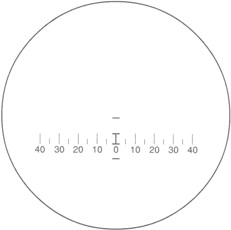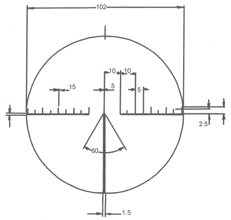## How to use the reticle

The reticle is a thin metal coated glas plate with a scaled mapping on his surface, located at the image plane of an afocal optical system. The basic unit for the use of a reticle is the military angular measure called ´point´, which 1 point means about 1 meter at 1000 meters. The basic for the deduction of this military angular measure is the so called ´full artillery circle´, which is subdivided into 6400 points (NATO). This division is the result of the circle perimeter calculation of a circle with the radius of 1000 meters, the result is 2πr = 2 x 3.14159 x 1000 = 6283.18.  From a mathematical point of view the circle perimeter is the sum of its chords, in which the precision of the calculation is depending from the number of this chords. For sufficiently approximation and for easier calculation in right-angeled coordinate systems, the nearest integer divisible by 4 will be used - 6400 (NATO). The result for this simplification is 6283.18 / 6400 = 0.98174, rounded 1 point.

With this reference value and the reticle the approximate distance of an object or other equation values can be determined. The equation is as follows:

Known dimension of an object multiplied by 1000 and divided by the readed number of points results in the approximate distance of the object.

For example, truck with the standard width of 2.50 meters:
If the truck fits 2 of the bigger points on the reticle, this is called ´0-10´ and it can be calculated 2.50 x 1000 / 10 = 250, resulting in a distance of 250 meters of the truck.

In former warsaw pact states the ´full artillery circle´ was suddevided into 6000 points, which means 6283.18 / 6000 = 1.04719, rounded also 1 point. The calculated difference per point is at 1000 meter about 6.5 cm, by what all these optical instrumantation have been useless!Source: Guido Thürnagel